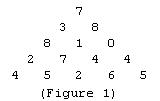favorite We need a little bit of your help to keep things running, click on this banner to learn more
Problems

# The TriangleFigure shows a number triangle. Write a program that calculates the highest sum of numbers passed on a route that starts at the top and ends somewhere on the base.

• Each step can go either diagonally down to the left or diagonally down to the right.
• The number of rows in the triangle is greater than 1 but less or equal 100.
• The numbers in the triangle, all integers, are between 0 and 99.

#### Input

First given the number of rows in triangle. Next rows describe the triangle itself.

#### Output

Print the maximum sum from top to the base of triangle.

Time limit 1 second
Memory limit 122.17 MiB
Input example #1
```5
7
3 8
8 1 0
2 7 4 4
4 5 2 6 5
```
Output example #1
```30
```
Source IOI-1994 Day1